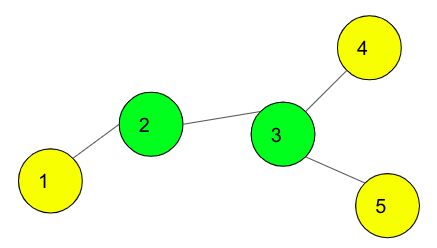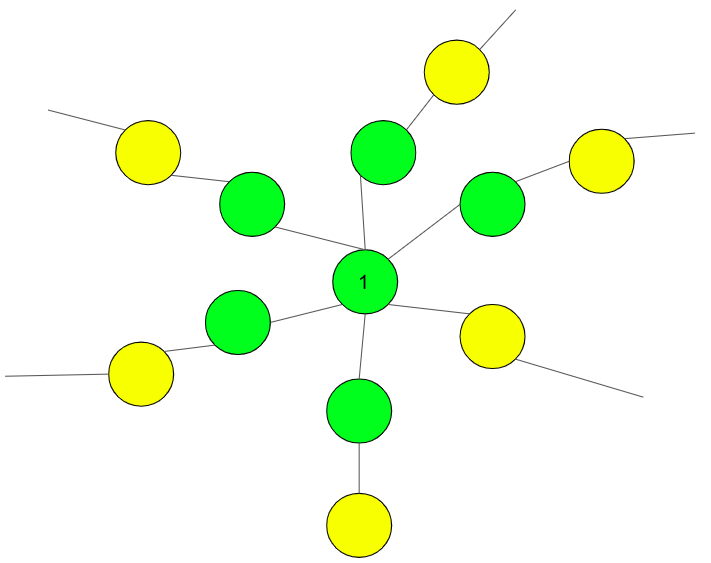Related Articles

# Tree with N nodes and K leaves such that distance between farthest leaves is minimized

• Last Updated : 05 May, 2021

Given N and K, print a tree such that the tree has no more than K leaf nodes and every other node has at least two nodes connected to it. The task is to build a tree of N nodes exactly in such a way that the distance between the farthest leaf nodes is minimized. Print the minimized distance also.
Note: There can be multiple trees.
Examples:

Input: N = 5, K = 3
Output: Distance = 3
The tree is:
1 2
2 3
3 4
3 5Input: N = 3, K = 2
Output: Distance = 2
The tree is:
1 2
2 3

Approach:

• Initially, the tree will have k-1 nodes connected to 1.
• Then connect one node to all the k-1 nodes one by one.
• If nodes are left, keep connecting them to the leaf nodes one by one.

The diagrammatic representation of how to build the tree will make things more clear. In the image below, K = 6 and for any number N has been demonstrated. The nodes in yellow are the leaf nodes.Below is the implementation of the above approach:

## C++

 `// C++ program of above approach``#include ``using` `namespace` `std;` `// Function to print the distance``// and the tree``void` `buildTree(``int` `n, ``int` `k)``{``    ``int` `ans = 2 * ((n - 1) / k) + min((n - 1) % k, 2);``    ``cout << ``"Distance = "` `<< ans;` `    ``cout << ``"\nThe tree is:\n"``;` `    ``// print all K-1 leaf nodes attached with 1``    ``for` `(``int` `i = 2; i <= k; i++) {``        ``cout << ``"1 "` `<< i << endl;``    ``}` `    ``// Join nodes to from other left nodes``    ``// the last node thus will be the left out leaf node``    ``for` `(``int` `i = k + 1; i <= n; i++) {``        ``cout << i << ``" "` `<< (i - k) << endl;``    ``}``}` `// Driver Code``int` `main()``{``    ``int` `n = 5, k = 3;` `    ``buildTree(n, k);``}`

## Java

 `// Java program of above approach``import` `java.util.*;``import` `java.lang.*;` `// Function to print the distance``// and the tree``class` `GFG``{``public` `void` `buildTree(``int` `n, ``int` `k)``{``    ``int` `ans = ``2` `* ((n - ``1``) / k) +``            ``Math.min((n - ``1``) % k, ``2``);``    ``System.out.println(``"Distance = "` `+ ans);` `    ``System.out.println(``"The tree is: "``);` `    ``// print all K-1 leaf nodes``    ``// attached with 1``    ``for` `(``int` `i = ``2``; i <= k; i++)``    ``{``        ``System.out.println( ``"1 "` `+ i );``    ``}` `    ``// Join nodes to from other left``    ``// nodes the last node thus will``    ``// be the left out leaf node``    ``for` `(``int` `i = k + ``1``; i <= n; i++)``    ``{``        ``System.out.println( i + ``" "` `+``                        ``(i - k));``    ``}``}` `// Driver Code``public` `static` `void` `main(String args[])``{``    ``GFG g = ``new` `GFG();``    ``int` `n = ``5``, k = ``3``;` `    ``g.buildTree(n, k);``}``}` `// This code is contributed``// by Akanksha Rai(Abby_akku)`

## Python3

 `# Python3 program of above approach` `# Function to print the distance``# and the tree``def` `buildTree(n, k):` `    ``ans ``=` `(``2` `*` `((n ``-` `1``) ``/``/` `k) ``+``            ``min``((n ``-` `1``) ``%` `k, ``2``))``    ``print``(``"Distance = "``, ans )` `    ``print``(``"The tree is:"``)` `    ``# print all K-1 leaf nodes``    ``# attached with 1``    ``for` `i ``in` `range``(``2``, k ``+` `1``):``        ``print``(``"1 "``, i)``    ` `    ``# Join nodes to from other left nodes``    ``# the last node thus will be the``    ``# left out leaf node``    ``for` `i ``in` `range``(k ``+` `1``, n ``+` `1``):``        ``print``(i, "", (i ``-` `k))` `# Driver Code``if` `__name__ ``=``=` `'__main__'``:``    ``n ``=` `5``    ``k ``=` `3``    ``buildTree(n, k)``    ` `# This code is contributed``# by SHUBHAMSINGH10`

## C#

 `// C# program of above approach``using` `System;` `// Function to print the distance``// and the tree``class` `GFG``{``public` `void` `buildTree(``int` `n, ``int` `k)``{``    ``int` `ans = 2 * ((n - 1) / k) +``        ``Math.Min((n - 1) % k, 2);``    ``Console.WriteLine(``"Distance = "` `+ ans);` `    ``Console.WriteLine (``"The tree is: "``);` `    ``// print all K-1 leaf nodes``    ``// attached with 1``    ``for` `(``int` `i = 2; i <= k; i++)``    ``{``        ``Console.WriteLine( ``"1 "` `+ i );``    ``}` `    ``// Join nodes to from other left``    ``// nodes the last node thus will``    ``// be the left out leaf node``    ``for` `(``int` `i = k + 1; i <= n; i++)``    ``{``        ``Console.WriteLine ( i + ``" "` `+``                        ``(i - k));``    ``}``}` `// Driver Code``public` `static` `void` `Main()``{``    ``GFG g = ``new` `GFG();``    ``int` `n = 5, k = 3;` `    ``g.buildTree(n, k);``}``}` `// This code is contributed by Soumik`

## PHP

 ``

## Javascript

 ``
Output:
```Distance = 3
The tree is:
1 2
1 3
4 1
5 2```My Personal Notes arrow_drop_up